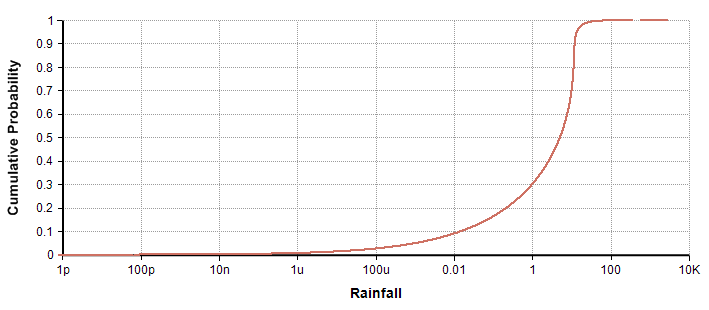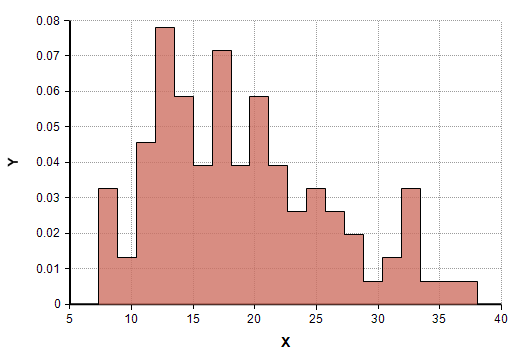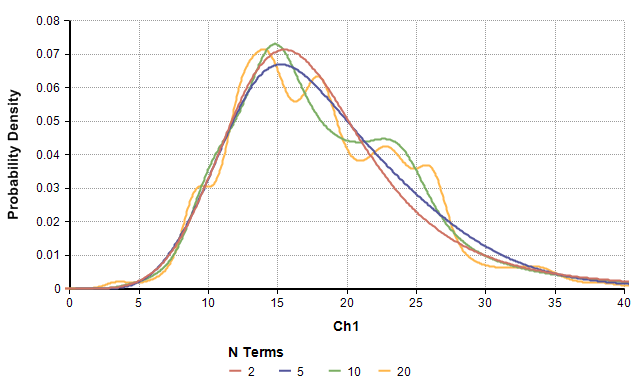# Keelin (MetaLog) distribution

(Redirected from Keelin)

New to Analytica 5.0

## Keelin( values , percentiles, I, lb, ub, nTerms, flags, over)

The Keelin distribution, also known as the "MetaLog" distribution, is a remarkably flexible distribution capable of taking on the shape of almost all common continuous distributions. It fits a distribution to specified fractile «values» with specified probability «percentiles» and optional lower and upper bound, «lb» and «ub». For the common special case, when you have three fractiles with symmetric probabilities -- for example 10, 50, and 90th percentiles where 10 and 90 are symmetrical around 50 -- it is more convenient to use the UncertainLMH() function. The Keelin distribution is also a convenient way to fit a probability distribution to a random sample of data, without having to worry about what parametric form is best.

The Keelin distribution is among the most versatile of all distributions, with an ability to produce unbounded, semi-bounded and bounded distributions, including uniform, beta, normal, gamma, with nearly any theoretically possible combination of skewness and kurtosis. In this respect, it is even more flexible that the family of Pearson distributions. Tom Keelin introduced the distribution in:

You can specify a Keelin distribution in three ways:

• From a list of (value, percentile) pairs, where the «values» are sample values for the quantity and the «percentiles» are the percentile (aka quantile or fractile) probabilities between 0 and 1. Another way of saying this is that «values», «percentiles» are points on the cumulative probability curve.
• From a set of representative points (a data sample).
• From a coefficient vector. These coefficients are typically obtained from data «values», or from (value, percentile) pairs using the function KeelinCoefficients. The coefficient vector may be a must shorter description of the distribution than the original data. When passing coefficients, you must specify the «flags»=1 bit, and then «I» index indexes the basis terms rather than the data.

### Parameters

• «values»: This parameter may be either (1) a random sample of data points from a distribution, with «percentiles» omitted, (2) a collection of fractile or percentile values (corresponding to the probability levels in «percentiles»), or (3) a Keelin coefficient vector with the «flags»=1 bit set. In all cases, «values» must be indexed by «I».
• «percentiles»: (Optional): The percentile probabilities (also called quantile or fractile levels) for the values in «values», also indexed by «I». Each number must be between 0 and 1. For example, when a value in «percentiles» is 0.05, the corresponding value in «values» is the 5th percentile.
• «I»: (Optional): The index of «values» and «percentile». You can omit this index when either «values» or «percentile» is itself an index.
• «lb», «ub»: (Optional) Upper and lower bound. Set one or both of these to a single number if you know in advance that your quantity is bounded. When neither is specified, the distribution is unbounded -- i.e., with tails going to -INF and INF. When one is set the distribution is semi-bounded, and when both are set it is fully bounded.
• «nTerms»: (Optional) The number of basis terms used for the fit. This should be 2 or greater. See #Number of terms below.
• «flags»: (Optional) A bit-field, where any of the following flags can be added together.
1: «values» contains coefficients (as obtained from the KeelinCoefficients function). When not set, «values» contains sample values.
8: Do not issue a warning when the Keelin distribution is infeasible. (See Infeasibility below). It does not test feasibility when «values» are Keelin coefficients -- i.e., when «flags»=1.
16: Return a sample even when infeasible. When this bit is not set, Null is returned if infeasible. When this is set, a mid-value or sample is returned anyway.
32: Disable tail constraints. By default, the Keelin function uses tail constraints to reduce the frequency of infeasible, an improvement to the algorithm published in the original Keelin paper. You can disable these to reproduce the original Keelin algorithm. See Infeasibility for details.
• «over»: (Optional) A list of indexes to sample independently across.

See also the analytic functions for the Keelin distribution (see Analytic distribution functions below):

CumKeelinInv( p, values, percentiles, I, lb, ub, nTerms, flags)
CumKeelin( x, values, percentiles, I, lb, ub, nTerms, flags)
DensKeelin( x,p, values, percentiles, I, lb, ub, nTerms, flags)

## Examples

### Fit to data

Suppose you've collected data on the weights of fish caught last year in the Columbia river, and now you want to fit a distribution to these measurements. Since you know that a fish's weight cannot be negative, you'll use a semi-bounded distribution. Suppose the data is in a variable named Fish_weight which in indexed by Fish_ID. Use

Keelin( Fish_weight, I:Fish_ID, lb: 0)

### Using fractiles

You find a published table stating the 500-year, 100-year, 10-year and median rain fall levels for a town of interest (where the 500-year level is a level so big that it is experienced only once every 500 years).

Index Fractile := [ 1/2, 1/10, 1/100, 1/500 ]
Variable Rainfall_level := Table(Fractile)(5, 12, 25, 60)
Chance Rainfall := Keelin( Rainfall_level, Fractile, lb:0 )

The resulting CDF is plotted here on a log-X scale:## Infeasibility

Some combinations of «values», «percentiles» and «nTerms» are considered to be infeasible. These are situations in which the Keelin algorithm cannot find a sensible distribution function that fits the data. See Keelin (2016) for mathematical details.

Infeasible data usually has sharp changes in slope or inconsistencies in monotonicity. If you plot your data as «values» vs. «percentiles» (or as «values» verses the rank of the data point when «percentiles» is omitted, the points should ideally be arranged in a monotonically increasing order. When there are many exceptions to this, then your percentile estimates are nonsense, which will often cause infeasibility. When data points "clump", this can result in rapid changes to the inferred slope of this graph, and can also contribute to infeasibility. Both these effects are generally lessened by decreasing «nTerms». When «nTerms» is much less than the number of data points, the fitting algorithm is better able to adapt to random fluctuations. The effect is to bias the fit away from distributions with too much variation.

By default, when it fits a Keelin distribution to the data, it tests whether the fit is feasible. This test is not performed when coefficients are passed in, but the test for feasibility will have been performed by KeelinCoefficients when that function fit the data. The test for feasibility can be skipped by setting the «flags»=8 bit. If the fit is found to be infeasible, a warning is issued (unless you have warnings suppressed) and the function returns Null. If either of the «flags»=8 or «flags»=16 bits is set, then it returns a sample even when infeasible. In theory, a sample from an infeasible fit is not guaranteed to match the data, but in practice it still tends to be pretty good. However, the results from the analytic functions such as CumKeelinInv, CumKeelin and DensKeelin don't make sense (specifically, CumKeelinInv is not monotonically increasing, and DensKeelin has negative values).

The algorithm used by Analytica includes an enhancement that expands the number of feasible cases compared to the original algorithm in Keelin (2016) . During the data fit, it adds the constraints that the left tail of the distribution should go left and the right tail should go right. For any valid distribution this obvious condition must hold. The condition does not guarantee it will find a feasible fit, but by enforcing this constraint the algorithm is able to locate many feasible solutions that it otherwise would not be able to find. This extension can be disabled by setting the «flags»=32 bit. The only reason we can think of for why you might want to disable it would be if you needed to reproduce the exact behavior of the original Keelin algorithm, perhaps if you are comparing results from a different implementation. But feasible solutions for the original Keelin algorithm are unaffected by tail constraints, so it would only be relevant if you needed to reproduce a nonsense case.

## Number of terms

The optional «nTerms» parameter varies the number of basis terms used for the fit. A larger number of terms results in a more detailed fit, but may also overfit when the data has randomness. Varying «nTerms» may also impact whether the data is or is not infeasible.

With 2 terms, the smallest that should be considered, the distribution is limited to a Logistic distribution (or Log-Logistic or Logit-Logistic when «lb» or «ub» are set), which gives it enough flexibility to match mean and standard deviation, but not skewness or kurtosis. With 3 terms skewness can be adjusted, but not kurtosis. With 4 terms, the median, variance, skewness and kurtosis can all be adjusted. In most cases, increasing «nTerms» enables it to fit your target distribution more closely.

You may find it useful to create a panel of fit distributions by varying «nTerms», making it possible to see what detail is revealed by the addition of terms, and also where the addition of more terms doesn't add useful detail. In many cases I've observed that there is an improved "fit" up to a point, followed by a plateau with very little change as «nTerms» increases, eventually followed by obvious over-fitting where it starts capturing the random spacing of samples. Often the plateau lasts for a long time. In these cases, it would make sense to set «nTerms» to a value on the plateau.

To create a "panel", first create an index: Index NumTerms := 2..50

Then use your data (say x indexed by I) to explore these: Variable fit_x :=  Keelin( x, ,I, nTerms:NumTerms)

In the following experiment, a data set with 100 measurements (not from any know distribution) was fit. A histogram of the data itself is shown here:Here are four "fit" Keelin distributions as «nTerms» was varies from 2 to 20:At 10 terms, a bi-modal effect starts to appear, which may actually be there in the data, and which is not visible below 10 terms, However, at 20 terms there appears to be more variation than is probably warranted, which we might interpret as the onset of overfitting.

## Analytic distribution functions

The Keelin function is a distribution function, meaning that it returns the median when evaluated in Mid-mode, a sample for Monte Carlo analysis when evaluated in Sample-mode, or a random variate when it is used in Random(). There are also analytic distribution functions for Keelin described in this section. The analytic distribution functions compute the exact value for a given Keelin without sampling error. These use the same parameters as Keelin( ), but also include a point of percentile where the analytic function is to be evaluated at, and this parameter comes first. It is common to pass an array of values in for the point or percentile parameter.

### CumKeelinInv( p, values, percentiles, I, lb, ub, nTerms, flags)

The inverse cumulative distribution function, also called the quantile function. Given a percentile (quantile) level «p», returns the value x such that there is a «p» probability that the actual value (or a value sampled at random from the distribution) is less than or equal to x.

Given a data set xi indexed by I, the 10-25-50-75-90 percentiles are obtained using:

Index percent=[10%, 25%, 50%, 75%, 90%] Do CumKeelinInv( percent, xi, , I)

Passing a Uniform(0,1) for «p» is equivalent to the Keelin distribution function:

CumKeelinInv( Uniform(0,1), xi )

### CumKeelin( x, values, percentiles, I, lb, ub, nTerms, flags)

The cumulative distribution function. For a given value «x», returns the probability that the true value (or a value sampled at random from the distribution) is less than or equal to «x».

Index quatile := [10%, 25%, 50%, 75%, 90%]
Index estimate := Table(quantile)( 20, 40, 70, 100, 130 )

This returns the probability that the quantity is less than 50:

CumKeelin( 50, estimate, quantile, quantile) → 0.33

### DensKeelin( x,p, values, percentiles, I, lb, ub, nTerms, flags)

The probability density function. Either «x» or «p», but not both, must be specified. When «x» is specified, returns the probability density at «x». When «p» is specified, returns the probability density at the «p»th quantile.

Using quantile and estimate shown in the example for CumKeelin above,

DensKeelin( 50, values:estimate, percentiles:quantile, I:quantile ) → 8.16m
DensKeelin( p:33%, values:estimate, percentiles:quantile, I:quantile ) → 8.16m

A good way to graph the probability density function is to create an X-Y plot of DensKeelin versus CumKeelinInv. This is illustrated here, again using the quantile and estimate example from above.

Index p := Sequence(0.1%, 99.9%, 0.1%);
Index K := 1..5;
Local a := KeelinCoefficients( estimate, quantile, quantile, K );
Local x := CumKeelinInv( p, a, I:K, flags:1 );
Local density := DensKeelin( p:p, values:a, I:K, flags:1 );
Index AxisLabel := ['X', 'Probability Density'];
Array( AxisLabel, [x,density] )


You need to plot this as an X-Y chart by pressing the [XY] button in the result window and enabling Use a comparison index. The comparison index here will be .AxisLabel.In the graph, an efficiency is obtained by calling KeelinCoefficients so that the data fit is performed only once, so that it doesn't have to be repeated in both successive calls to CumKeelinInv and DensKeelin. It evaluates both at 999 points (the number of points in p. Zero and 1 are not included in p since these would be -Inf and Inf in a distribution with tails like this. A nice thing about this approach is that it is easy to vary over the full range of p without having to know the actual range of the quantity itself.